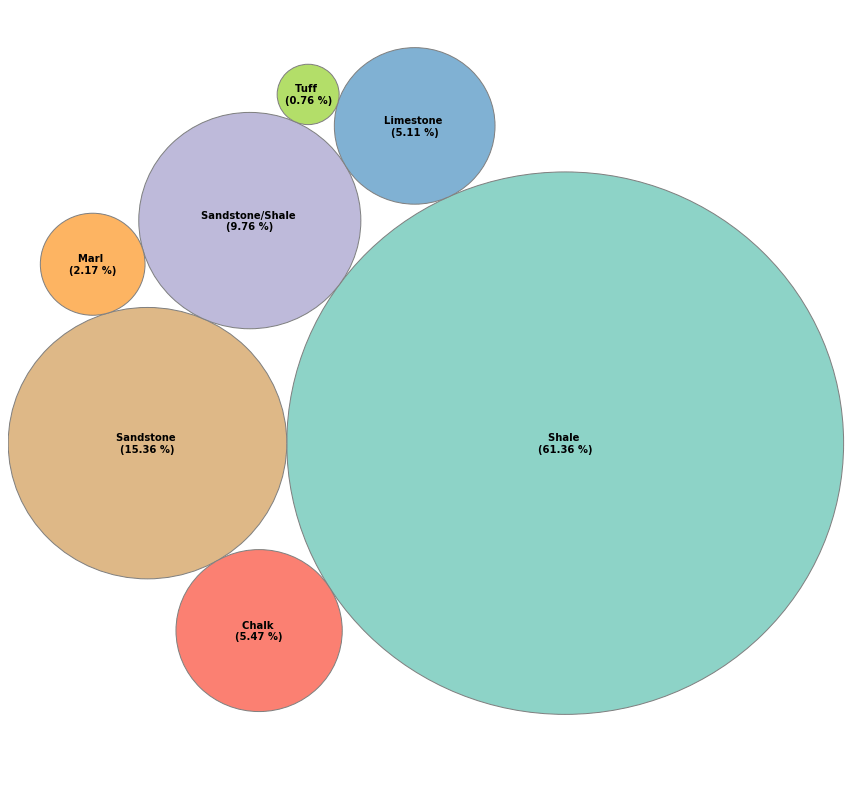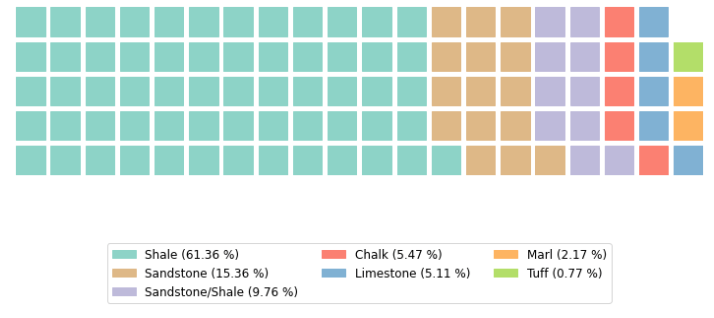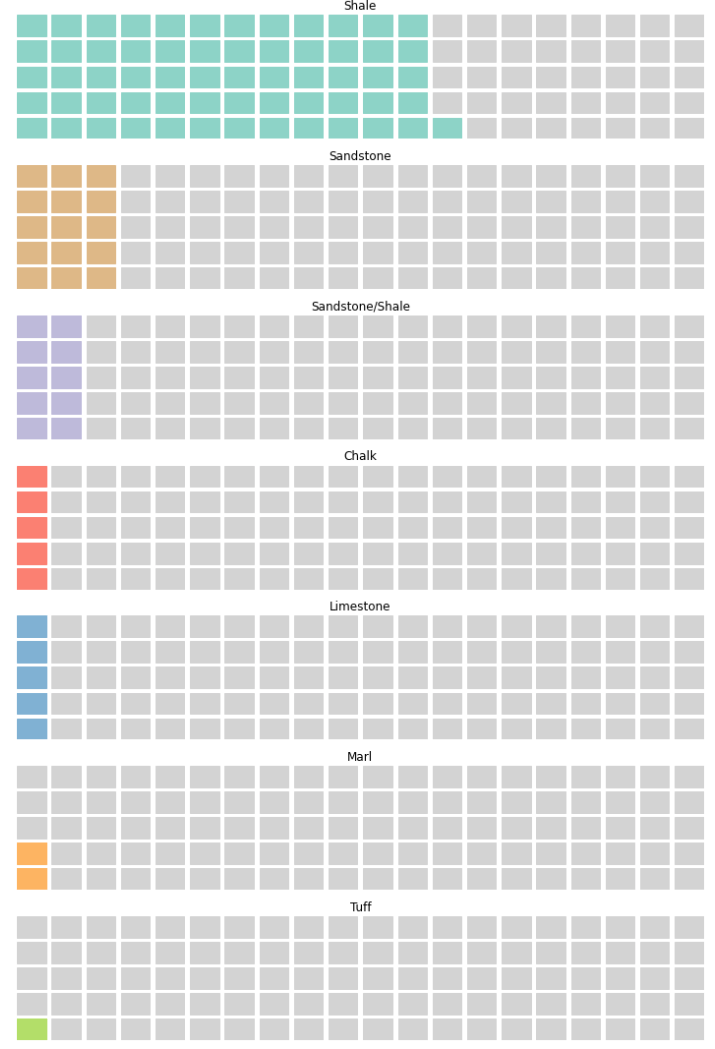# 9 Creative Alternatives to the Traditional Pie Chart for Data Visualisation

Pie charts are a commonly used, easy to create circular graphic for visualising the relative sizes of different categories that contribute to a whole. Each slice within the pie chart represents a category, and its size is relative to its contribution. They are useful visualisations when dealing with a limited number of categories.

Even though pie charts are a common data visualisation, there are several disadvantages to using them, including:

• Humans are not naturally great at estimating quantities from angles
• They can become overcrowded when a large number of categories are used
• Small portions/percentages are hard to visualise accurately
• Hard to compare multiple pie charts
• Hard to interpret when the charts are made to look 3D

For more information on some of the issues experienced with pie charts, check out this section on Wikipedia.

Within this article, we are going to see how to create 9 different alternatives to pie charts using Python.

If you want to recreate the visualisations on this page, you will need to import a few essential libraries and create a dummy dataset. If you have your own dataset, you can skip the data creation section and reference your own data.

The first step we will need to carry out is importing the main libraries we will be working with. These are pandas for loading and working with our data, matplotlib to create the plots and numpy for some basic data manipulation.

`import pandas as pdimport matplotlib.pyplot as pltimport numpy as np`

Next, we will create our dataframe from a dictionary. For this example, I am using a very simple dataset. It consists of 7 different lithologies (rock types) and their associated percentages. Each percentage represents how much of that lithology is present within a specific geological formation or well.

This is a very simplistic dataset, and real datasets can be much more varied.

`lith_dict = {'LITH': ['Shale', 'Sandstone',                       'Sandstone/Shale', 'Chalk',                       'Limestone', 'Marl', 'Tuff'],             'PERCENTAGE': [61.36,15.36, 9.76, 5.47,                             5.11, 2.17, 0.77]}lith_data_df = pd.DataFrame.from_dict(lith_dict)`

When we display the `lith_data_df` dataframe, we get the following:

We will also setup some colours to keep the plots consistent as we go through the article.

`colours = ['#8dd3c7', '#deb887', '#bebada', '#fb8072',            '#80b1d3', '#fdb462', '#b3de69']`

# Creating Pie Charts in Python

Now that the data has been loaded, let us look at the data in a pie chart. This is easily done using matplotlib as follows.

`lith_labels = lith_data_df['LITH'].unique()plt.figure(figsize=(10,10))plt.pie(lith_data_df['PERCENTAGE'],         labels=lith_labels,         colors=colours,         startangle=90,         wedgeprops={"linewidth": 1, "edgecolor": "grey"})plt.show()`

We now have our first pie chart. We can see some of the issues starting to appear.

We can see that the Chalk and Limestone slices are very similar in size and could be considered equal. Also, the Tuff lithology slice is very small, and we may not be able to estimate it’s size accurately.

To help improve the pie chart, we could add percentage labels to help the reader understand how much each slice represents.

`lith_labels = lith_data_df['LITH'].unique()plt.figure(figsize=(10,10))pie_chart = plt.pie(lith_data_df['PERCENTAGE'],                     labels = lith_labels,                     colors=colours,                    startangle=90,                    autopct='%0.1f%%',                      wedgeprops={"linewidth": 1, "edgecolor": "grey"},                    pctdistance=0.5)plt.show()`Matplotlib pie chart showing different proportions of lithologies with percentage values labelled. Image by the author.

Now that we have percentage labels displayed, we can understand how much each slice represents. However, we can see that as soon as we have multiple smaller slices, we start to get overlapping labels.

# Alternatives to Pie Charts

There are several alternatives to pie charts that you can easily and quickly create with Python. Let’s have a closer look at them.

# 1. Donut Chart

A popular alternative to pie charts is the donut chart. These are essentially pie charts with a great big hole in the middle.

Each category is represented by an arc rather than a slice. This allows the reader to focus on the length of the arc rather than the area, angle and size of the slices. Additionally, this can help lead the reader’s eye around the different groups and improve the narrative of the story being told by the data.

The hole in the centre can be left blank, but oftentimes a graphic or a number can be placed there to help with the storytelling.

However, they still have similar drawbacks to a pie chart, including if too many categories are present or if the segments are very small.

We can modify the pie chart above and turn it into a donut chart.

`# Set up the plot labelsplot_labels = [f'{i} \n({str(j)} %)' for i,j in zip(lith_data_df.LITH,                                                     lith_data_df.PERCENTAGE)]plt.figure(figsize=(10,10))plt.pie(lith_data_df['PERCENTAGE'],         labels = plot_labels,        colors=colours,         startangle=90,         wedgeprops={"linewidth": 1, "edgecolor": "white"},         labeldistance=1.15)# Add inner circle and outer border to the donut chart# Allows us to have white seperations between the segmentscentre_circle = plt.Circle((0, 0), 0.70, fc='white', ec='grey')outer_circle = plt.Circle((0, 0), 1.00, fc='None', ec='grey')fig = plt.gcf()# Adding the circles to the chartfig.gca().add_artist(centre_circle)fig.gca().add_artist(outer_circle)plt.show()`

When we run the above code, we get back the donut plot. It does look better than the pie chart, and it is easier to get a feel for the size of each of the segments.

We still have issues with overlapping labels, but this could be resolved with a little more code or by switching to a legend.

# 2. Bar Charts

The first alternative to pie charts and donut charts people think of is the good old fashioned bar chart. They are easy to create and simple for the user to understand.

It is simple to display each slice of the pie chart as a single vertical (or horizontal bar if using a horizontal bar chart), where the height of the bar reflects the size of the slice. This makes it easy to show the relative sizes of each category.

They are also great for showing a larger number of categories compared to a pie chart, and you can avoid most issues that may arise from labelling.

One of the downsides of using a bar chart is it can be difficult to understand how each of the categories contributes to the whole.

We can easily create a bar chart with matplotlib as follows:

`plt.figure(figsize=(10,10))plt.bar(x=lith_data_df['LITH'], height=lith_data_df['PERCENTAGE'], color=colours)plt.xlabel('Percentage', fontsize='15', fontweight='bold', labelpad=30)plt.ylabel('Country', fontsize='15', fontweight='bold', labelpad=30)plt.show()`

When we run the above code, we get the following bar chart.

There are numerous suggestions for creating and styling bar charts, such as avoiding too many colours and ensuring that the y-axis starts from zero.

Check out the excellent article below on ways to improve how you present data with bar charts and subsequently improve readability for the user.

visage.co

# 3. Stacked Bar Charts

Stacked bar charts can be viewed as a linear version of the donut chart. From this, you can easily get an understanding of how each category contributes to the overall picture.

They allow us to display a large number of categories within a small space. It is also easier for us to understand size relationships with rectangular shapes than it is with angles and slices of a circle.

However, it can become difficult to compare different categories with each other if they are not the first in the series. They can also become cumbersome when the number of categories increases significantly.

We can create the stacked bar chart in matplotlib as follows.

`lith_data_df[['PERCENTAGE']].T.plot.barh(stacked=True,                                        legend=True, figsize=(15,2),                                         color=colours, edgecolor='grey')plt.axis('off')plt.legend(lith_data_df['LITH'].unique(), loc='lower center',            ncol = 7, bbox_to_anchor=(0.5, -0.2), frameon=False)plt.show()`

Notice that this code uses the `PERCENTAGE` column from the dataframe and transposes it first before using the `.plot` method from pandas.

To avoid any issues with any overlapping text, we can display a legend at the base of the figure with each of the categories.

From the generated chart, we can easily see that shale is the largest component of the chart, with Tuff being the smallest. However, if we want to start comparing Limestone, Chalk and Sandstone/Shale categories, it can be difficult without actual values.

# 4. Lolipop Chart

Lolipop charts are similar to bar charts, where the bar is substituted for a line, and a dot represents the end of the bar.

They are a good way to show variations between different categories — similar to bar charts — especially when you have a large number of categories. They can also be helpful when you have several categories with high and similar values.

One issue with a lollipop chart is it can be harder — compared to a bar chart — to obtain an accurate value, as the centre of the dot can be hard to identify.

To create a lollipop chart with matplotlib we can use the stem plot, which is simple to use; however, the formatting of it with this method can be limited.

`plt.figure(figsize=(10,5))plt.stem(lith_data_df['PERCENTAGE'])plt.grid(color='lightgrey', alpha=0.5)plt.xticks(ticks=range(0,len(lith_data_df)), labels=lith_data_df['LITH'])plt.xlabel('Lithology', fontsize=14, fontweight='bold')plt.ylim(0, 100)plt.ylabel('Percentage', fontsize=14, fontweight='bold')plt.show()`

If we want to add a bit of character to a lollipop chart, we can create one from scratch in matplotlib. This uses a combination of a scatter plot and vertical lines. Doing it this way allows us to control the colour of the stems and marker style.

`plt.figure(figsize=(10,5))plt.scatter(lith_data_df['LITH'], lith_data_df['PERCENTAGE'],             c=colours, s=100, edgecolors='grey', zorder=3)plt.vlines(lith_data_df['LITH'], ymin=0, ymax=lith_data_df['PERCENTAGE'],            colors=colours, linewidth=4, zorder=2)plt.ylim(0, 100)plt.ylabel('Percentage', fontsize=14, fontweight='bold')plt.xlabel('Lithology', fontsize=14, fontweight='bold')plt.grid(color='lightgrey', alpha=0.5, zorder=1)plt.show()`

Radar charts, also known as spider charts or star charts, are a way to display three or more variables within a 2-dimensional chart. The data are plotted on the chart in a circular layout, with each variable represented as a spoke that extends from the centre of the chart. The position of the variable along the spokes provides an indication of the magnitude of that variable represented by that spoke.

Radar plots can be created using matplotlib like so:

`lithologies = list(lith_data_df['LITH'])percentages = list(lith_data_df['PERCENTAGE'])lithologies = [*lithologies, lithologies]percentages = [*percentages, percentages]label_loc = np.linspace(start=0, stop=2 * np.pi, num=len(lithologies))plt.figure(figsize=(10,10))plt.subplot(polar=True)plt.plot(label_loc, percentages, lw=4)lines, labels = plt.thetagrids(np.degrees(label_loc), labels=lithologies)plt.plot()plt.show()`

Alternatively, a more interactive version can be created using Plotly Express.

`import plotly.express as pxfig = px.line_polar(lith_data_df,                     r='PERCENTAGE',                    theta='LITH',                     line_close=True,                    width=800,                    height=800)fig.update_traces(fill='toself', line = dict(color='red'))fig.show()`

Radial bar charts are essentially bar charts that have been plotted on a polar co-ordinate system. Instead of the bars extending vertically or horizontally from an axis, they extend radially from the centre of the chart. They are a great way to visualise data that is cyclical in nature and can be visually interesting to the reader.

When using a radial bar chart, it can be hard to compare categories that are not adjacent to each other directly.

Using python and some simple maths, we can place each category/bar around the plot and display it using matplotlib.

`labels = lith_data_df['LITH'].unique()fig, ax = plt.subplots(subplot_kw={'projection': 'polar'}, figsize=(10,10))angles = np.linspace(0, 2*np.pi, len(lith_data_df), endpoint=False)upper_limit = 100lower_limit = 0max_value = lith_data_df['PERCENTAGE'].max()indexes = list(range(0, len(lith_data_df)))angles = [element * width for element in indexes]width = 2*np.pi / len(lith_data_df)# Create the barsbars = ax.bar(x = angles, height=lith_data_df['PERCENTAGE'], width=width,        color=colours, edgecolor='black', zorder=2, alpha=0.8)plt.grid(zorder=0)# Remove all ticks and labels from x & y axis but keep border onplt.tick_params(axis='x', which='both', bottom=False, left=False,                labelbottom=False, labelleft=False)# Control the scale of the circleplt.ylim(0, 70)ax.legend(bars, labels, loc='center right', bbox_to_anchor=(1.3, 0.5))plt.show()`

When the above code is run, we are presented with the following chart. We can see the dominance of shale within the chart and the smaller contributions of the other lithologies. Also, we can see that it can be difficult to visualise categories, like Tuff, in this kind of plot.

# 7. Treemaps

Treemaps are a simple alternative to pie charts and were developed in the 1990s by Ben Shneiderman. They are a rectangle or square made up of smaller rectangles, where the size of each sub-rectangle is proportional to the size of the data it represents. Commonly, they are used to represented hierarchical datasets and can be used with a large amount of data.

To create treemaps in Python, we can call upon the squarify library. This library makes creating tree maps very simple.

`import squarify# Set up the plot labelsplot_labels = [f'{i} \n({str(j)} %)' for i,j in zip(lith_data_df.LITH,                                                     lith_data_df.PERCENTAGE)]plt.figure(figsize=(10,10))squarify.plot(sizes=lith_data_df['PERCENTAGE'],               label=plot_labels, color=colours, edgecolor='grey')# Remove all ticks and labels from x & y axis, but keep border onplt.tick_params(axis='both', which='both', bottom=False, left=False,                labelbottom=False, labelleft=False)plt.show()`

As you can see, the code above is very simple, but it generates a powerful and visually appealing chart.

We can immediately see the dominance of the Shale lithology within our dataset. If you have a large number of categories or a few small categories, then one of the issues that could arise from using tree maps is not being able to identify what some of the squares represent. This is where some interactivity would be extremely beneficial.

Treemaps also have several disadvantages, with one being that humans are poor at judging relative sizes and areas. This can become more of an issue when we have a large number of categories to display.

# 8. Packed Circle Chart / Circular Treemap

Instead of using squares and rectangles to represent each of the categories like a treemap, we can use circles. A packed circle chart can be a great way to display large amounts of data in a visually appealing way.

Although, they are not great if you need to compare precise values between each of the categories. They are also affected by the issue of humans not being able to compare different sized areas accurately.

To generate a Circle Chart in Python, we can combine the circlify library with matplotlib.

When `circlify.circlify` is called upon, it generates an array that is arranged from the smallest value to the largest. To match up the right circle with the correct label and colour, we need to reverse the array.

`import circlifycolours = ['#8dd3c7', 'burlywood', '#bebada', '#fb8072',            '#80b1d3', '#fdb462', '#b3de69']plot_labels = [f'{i} \n({str(j)} %)' for i,j in zip(lith_data_df.LITH,                                                     lith_data_df.PERCENTAGE)]circle_plot = circlify.circlify(lith_data_df['PERCENTAGE'].tolist(),                                target_enclosure=circlify.Circle(x=0, y=0))# Note that circle_plot starts from the smallest to the largest, # so we have to reverse the listcircle_plot.reverse()fig, axs = plt.subplots(figsize=(15, 15))# Find axis boundarieslim = max(max(abs(circle.x) + circle.r,               abs(circle.y) + circle.r,)           for circle in circle_plot)plt.xlim(-lim, lim)plt.ylim(-lim, lim)# Display circles.for circle, colour, label in zip(circle_plot, colours, plot_labels):    x, y, r = circle    axs.add_patch(plt.Circle((x, y), r, linewidth=1, facecolor=colour,                              edgecolor='grey'))    plt.annotate(label, (x, y), va='center', ha='center', fontweight='bold')plt.axis('off')plt.show()`

When we run the above code, we get back the following plot.Packed circle plot as an alternative to a pie chart. Image by the author.

# 9. Waffle Chart

Waffle charts are a visually appealing way to display categorical data. They are easy to interpret and can be used to create a good narrative for the reader to follow.

They look much better than pie charts and are not distorted; however, they really only should be used when we have a small number of categories. If we have a large number of categories in our dataset, we then end up with the same problem we have with pie charts, where they become unreadable.

Waffle charts are square or rectangular displays consisting of smaller squares set in a grid pattern. Each square within the grid is coloured based on a category and represents a portion of the whole. From these plots, we can see contributions of individual categories or display progress towards a goal.

`from pywaffle import Wafflefig = plt.figure(FigureClass=Waffle, figsize=(10,10), rows=4, columns = 20,                  values=list(lith_data_df['PERCENTAGE']),                 colors=colours,                 labels=plot_labels,                 legend={'loc':'lower center', 'bbox_to_anchor': (0.5, -0.8),                         'ncol':3, 'fontsize':12})plt.show()`

When we run the above code, we get the following Waffle chart.Waffle chart as an alternative to pie charts. Note the missing square in the top right, which is a result of rounding the values up and down to the nearest whole number. Image by the author.

One small issue with Waffle charts created by PyWaffle that you can see above is if you have numbers with decimal values, PyWaffle will attempt round those numbers to the nearest whole number. By default, this will be either up or down.

You can control the way the rounding is carried out by adding a parameter called `rounding_rule` and manually set it to floor, ceil or nearest. However, this may end up messing up the display and showing incorrect colouring.

Ideally, if our data sums to 100% after rounding, we should end up with a chart as follows.Example of a waffle chart showing different lithologies found within a well. Image by the author.

An alternative way of displaying data on waffle charts is by splitting up each category into its own chart. This is achieved using matplotlib and looping through each category, and creating a chart with `Waffle.make_waffle()`.

`off_colour = 'lightgrey'# Figsize numbers must be equal or the height greater than the width# othewise the plot will appear distortedfig, axs = plt.subplots(len(lith_data_df), 1, figsize=(10, 15))for (i, ax), color in zip(enumerate(axs.flatten()), colours):    plot_colours = [color, off_colour]    perc = lith_data_df.iloc[i]['PERCENTAGE']    values = [perc, (100-perc)]    lith = lith_data_df.iloc[i]['LITH']    Waffle.make_waffle(ax=ax, rows=4, columns=20,                        values=values, colors=plot_colours)        ax.set_title(lith)plt.tight_layout()plt.show()`Waffle chart for each category within our lithology dataset. Image by the author.

If you want to read more about Waffle charts, check out my other article below, which goes into them in more depth.

## How to Create Beautiful Waffle Charts for Data Visualisation in Python

### A Great Alternative to Pie Charts for Data Visualisation

towardsdatascience.com

# Summary

Pie charts can be useful to visualise datasets containing a small number of categories. However, they have several disadvantages. Within this article, we have covered 9 alternative charts which could be used instead, each with its own advantages and disadvantages

When creating effective data visualisations, it is important to consider your audience and the story you are trying to tell. This will allow you to select the most appropriate chart for the job.